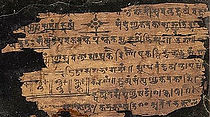top of page

# Kuttaka: Mathematical Elegance from Ancient IndiaTake a broad look at the Mathematics developed by the early Indian mathematicians/ astronomers, and dive deep into the Kuttaka method used to solve indeterminate equations with multiple solutions- the most elegant and effective method to do so, even today.

(This course is intended for Math enthusiasts Grade 9 and above i.e. high school students and grown-ups of any age)

In high school we learn to solve linear algebraic equations of one or two or three variables. We also learn to solve quadratic equations in one variable. We don’t learn solving indeterminate equations, which have multiple solutions.

A classic example of the above problem in what is known as Chinese Remainder Theorem, which is posed as follows: Find a number N which leaves a remainder 3 when divided by 7 and a remainder 1 when divided by 9. The most comprehensive generalised algorithm to solve such a problem was given by Aryabhata, in the 6th century CE. The solution method is known as Kuttaka.

These problems occur naturally in Astronomy and that is why the Indian mathematicians/astronomers were looking to solve them. For example, in a particular case of finding the number of days and the revolutions performed by the Sun, the following equation

y= (576x-86638)/ 210389

has to be solved for x, y as positive integers. It can be shown that the above astronomy problem and the remainder problem are one and the same. They end up in similar looking equations. To this day, Aryabhata’s method of solving them is the most elegant and efficient available.

We will take a broad look at the Mathematics developed by the early Indian mathematicians and then study the above problem in depth. We will solve such problems using modular arithmetic and then move to Aryabhata’s kuttaka algorithm.